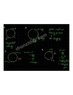# In the circular motion below, what will be the velocity?

• HCverma
It is not clear to me whether the use of complex variables to represent the vectors comes from the original problem or has been introduced by the student.f

## Homework Statement

In circular motion what will be the change in velocity if initial velocity and final velocity is 2 m/s? From the left, in the 3rd diagram, if initial velocity and final velocity is 2 m/ s then what is the change in velocity? t = 5 s

## The Attempt at a Solution

Acceleration = Vf - Vi / t = (-2i) - 2i / 5 = -4i / 5 = -0.8 m/s^2 but the answer is 0.8m/s^2.

#### Attachments

•circular-motion-2-1024.jpg
16.9 KB · Views: 343
I see two illegible drawings (pure black) and something in the middle with some semi-legible chicken scratchings.

I think you should re-state the problem and provide readable drawing, it is not clear to me. Hint: velocity is a vector, giving value like "2 m/s" is not sufficient.

•Delta2

## Homework Statement

In circular motion what will be the change in velocity if initial velocity and final velocity is 2 m/s? From the left, in the 3rd diagram, if initial velocity and final velocity is 2 m/ s then what is the change in velocity? t = 5 s

## The Attempt at a Solution

Acceleration = Vf - Vi / t = (-2i) - 2i / 5 = -4i / 5 = -0.8 m/s^2 but the answer is 0.8m/s^2.
I have edited the image so that you can understand. Please see the image and let me know.

#### Attachments

In circular motion what will be the change in velocity if initial velocity and final velocity is 2 m/s?
Acceleration = Vf - Vi / t = (-2i) - 2i / 5 = -4i / 5 = -0.8 m/s^2 but the answer is 0.8m/s^2.
The question asks for a change in velocity but the answer is an acceleration? That's wrong.

•SammyS
(-2i) - 2i

How can both velocity vectors have same unit vector?

According to your drawing the first one, one is along j and another along i.

The pictures are still not clear. What are you using?

How can both velocity vectors have same unit vector?

According to your drawing the first one, one is along j and another along i.

The pictures are still not clear. What are you using?
Could you suggest an online editor where I could sharpen my image as much clear as I can?

Yes, my question is that in the picture change in velocity is 4 or -4, As I know the change in velocity is Vf -Vi = (-2i) - 2i = -4i. please see the picture.
I would say that the change in velocity is neither 4 nor -4. It is -4i.

•HCverma
would you please let me know whether you could understand my edited image I provided so that in future I would edit my images to that degree. Is it readable?
It is not pretty, but it is magnified enough that I can read it.

•HCverma
It is not pretty, but it is magnified enough that I can read it.

I would say that the change in velocity is neither 4 nor -4. It is -4i.
It is not clear to me whether the use of complex variables to represent the vectors comes from the original problem or has been introduced by the student.
@HCverma , please state the problem as given to you, word for word.

•HCverma
It is not clear to me whether the use of complex variables to represent the vectors comes from the original problem or has been introduced by the student.
@HCverma , please state the problem as given to you, word for word.
Next time I will.

Next time I will.
Why not this time? As I understand it, you still lack an explanation for the book answer.

•jbriggs444